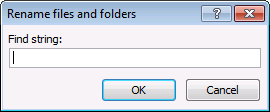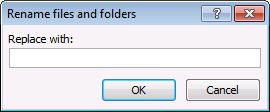Author: Oscar Cronquist Article last updated on July 29, 2017

The following two macros lets you rename files and folders recursively. Press Alt + F8 to open a list of macros, run macro "FindReplace".

1. Type a text string you want to find, in the dialog box.2. In the next dialog box, type a text sting you want to replace it with.3. Select a folder, click OK.
4. The macro prompts you each time it finds a file name or folder with the text string you are looking for, if you want to rename it.
5. Your options are: Yes, No and Cancel. Cancel exits the macro.
6. The macro continues until all files and folders are processed.

The macro does NOT change the file extension name.

### VBA macros

```Sub FindReplace()
Dim myfolder
Dim Fnd As String, Rplc As String
Fnd = Application.InputBox(prompt:="Find string:", Title:="Rename files and folders", Type:=2)
Rplc = Application.InputBox(prompt:="Replace with:", Title:="Rename files and folders", Type:=2)
With Application.FileDialog(msoFileDialogFolderPicker)
.Show
myfolder = .SelectedItems(1) &amp; "\"
End With
Call Recursive(myfolder, Fnd, Rplc)
End Sub
```
```Sub Recursive(FolderPath As Variant, Fnd As String, Rplc As String)
Dim Value As String, Folders() As String, Fname As String, Fext As String, Mtxt As String
Dim x As Integer
Dim Folder As Variant, a As Long
ReDim Folders(0)
If Right(FolderPath, 2) = "\\" Then Exit Sub
Value = Dir(FolderPath, &amp;H1F)
Do Until Value = ""
If Value = "." Or Value = ".." Then
Else
If GetAttr(FolderPath &amp; Value) = 16 Or GetAttr(FolderPath &amp; Value) = 48 Then
On Error Resume Next
Mtxt = "Rename folder " &amp; Value &amp; " to " &amp; WorksheetFunction.Substitute(Value, Fnd, Rplc) &amp; "?"
x = MsgBox(Mtxt, vbYesNoCancel)
If x = vbCancel Then Exit Sub
If x = vbYes Then
Name FolderPath &amp; Value As FolderPath &amp; WorksheetFunction.Substitute(Value, Fnd, Rplc)
End If
Value = WorksheetFunction.Substitute(Value, Fnd, Rplc)
If Err &lt;&gt; 0 Then
MsgBox "Error"
Exit Sub
End If
On Error GoTo 0
Folders(UBound(Folders)) = Value
ReDim Preserve Folders(UBound(Folders) + 1)
Else
On Error Resume Next
Fext = Split(Value, ".")(UBound(Split(Value, ".")))
Fname = Left(Value, Len(Value) - Len(Split(Value, ".")(UBound(Split(Value, ".")))) - 1)
Fname = WorksheetFunction.Substitute(Fname, Fnd, Rplc)
If Value &lt;&gt; (Fname &amp; "." &amp; Fext) Then
Mtxt = "Rename file " &amp; Value &amp; " to " &amp; Fname &amp; "." &amp; Fext &amp; "?"
x = MsgBox(Mtxt, vbYesNoCancel)
If x = vbCancel Then Exit Sub
If x = vbYes Then
Name FolderPath &amp; Value As FolderPath &amp; Fname &amp; "." &amp; Fext
End If
End If
If Err &lt;&gt; 0 Then
MsgBox "Error"
Exit Sub
End If
On Error GoTo 0
End If
End If
Value = Dir
Loop
For Each Folder In Folders
Call Recursive(FolderPath &amp; Folder &amp; "\", Fnd, Rplc)
Next Folder
End Sub
```